Most Affordable JEE | NEET | 8,9,10 Preparation by Kota's Top IITian Doctor Faculties

# Atomic Structure - JEE Main Previous Year Questions with SolutionsJEE Main Previous Year Question of Physics with Solutions are available here. Practicing JEE Main Previous Year Papers Questions of Physics will help all the JEE aspirants in realizing the question pattern as well as help in analyzing their weak & strong areas. Get detailed Class 11th &12th Physics Notes to prepare for Boards as well as competitive exams like IIT JEE, NEET etc. eSaral helps the students in clearing and understanding each topic in a better way. eSaral is providing complete chapter-wise notes of Class 11th and 12th both for all subjects. Besides this, eSaral also offers NCERT Solutions, Previous year questions for JEE Main and Advance, Practice questions, Test Series for JEE Main, JEE Advanced and NEET, Important questions of Physics, Chemistry, Math, and Biology and many more. Download eSaral app for free study material and video tutorials.   Simulator   Previous Years AIEEE/JEE Mains Questions
Q. The transistion from the state n = 4 to n = 3 in a hydrogen like atom results in ultraviolet radiation. Infrared radiation will be obtained in the transition from :- (1) $4 \rightarrow 2$ (2) $5 \rightarrow 4$ (3) $2 \rightarrow 1$ (4) $3 \rightarrow 2$ [AIEEE - 2009]
Ans. (2) Use Rydberg equation to find the required transition.
Q. Energy required for the electron excitation in $\mathrm{Li}^{++}$ from the first to the third Bohr orbit is: (1) 108.8 eV (2) 122.4 eV (3) 12.1 eV (4) 36.3 eV [AIEEE-2011]
Ans. (1) Rquired energy $=13.6(Z)^{2}\left[\frac{1}{n_{1}^{2}}-\frac{1}{n_{2}^{2}}\right]$ eV $=13.6(3)^{2}\left[\frac{1}{1^{2}}-\frac{1}{3^{2}}\right]=108.8 \mathrm{eV}$
Q. Hydrogen atom is excited from ground state to another state with principal quantum number equal to 4. Then the number of spectral lines in the emission spectra will be :- (1) 6 (2) 2 (3) 3 (4) 5 [AIEEE-2012]
Ans. (1) Number of lines $=^{n} C_{2}=^{4} C_{2}=\frac{(4)(3)}{(2)}=6$
Q. In a hydrogen like atom electron makes transition from an energy level with quantum number n to another with quantum number $(n-1) .$ If $n>>1$, the frequency of radiation emitted is proportional to : ( 1)$\frac{1}{\mathrm{n}}$ ( 2)$\frac{1}{\mathrm{n}^{2}}$ (3) $\frac{1}{\mathrm{n}^{3 / 2}}$ (4) $\frac{1}{n^{3}}$ [JEE Main-2013]
Ans. (4) Energy of $\mathrm{E}=\mathrm{h} \theta=\mathrm{E}_{0} \mathrm{z}^{2}\left[\frac{1}{(\mathrm{n}-1)^{2}}-\frac{1}{\mathrm{n}^{2}}\right]$ $=\mathrm{E}_{0} \mathrm{z}^{2}\left[\frac{2 \mathrm{n}-1}{\mathrm{n}^{2}(\mathrm{n}-1)^{2}}\right]$ $\mathrm{h} \theta \approx \mathrm{E}_{0} \mathrm{z}^{2}\left[\frac{2 \mathrm{n}}{\mathrm{n}^{4}}\right] \Rightarrow \mathrm{v} \propto \frac{1}{\mathrm{n}^{3}}$
Q. As an electron makes a transition from an excited state to the ground state of a hydrogen - like atom/ion : (1) kinetic energy decreases, potential energy increases but total energy remains same (2) kinetic energy and total energy decrease but potential energy increases (3) its kinetic energy increases but potential energy and total energy decreases (4) kinetic energy, potential energy and total energy decrease. [JEE Main-2015]
Ans. (3) $\mathrm{K}=+13.6 \frac{\mathrm{z}^{2}}{\mathrm{n}^{2}}$ as $\mathrm{n}$ decreases $\mathrm{k}$ increases
Q. Match List-I (Fundament Experiment) with List-II (its conclusion) and select the correct option from the choices given below the list :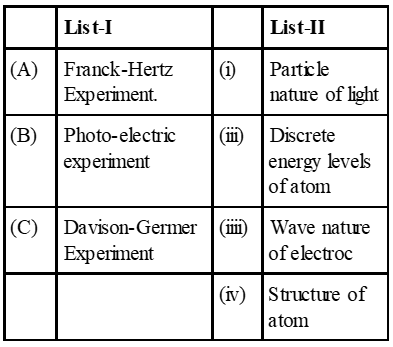(1) A-ii, B-i, C-iii (2) A-iv, B-iii, C-ii (3) A-i, B-iv, C-iii (4) A-ii, B-iv, C-iii [JEE Main-2015]
Ans. (1) Self Explanatory/Theory (A) Franck-Hertz experiment explains disrete energy levels of atom (B) Photo-electric experiment explain particle nature of light (C) Davison Germer experiment explain wave nature of electron.
Q. Some energy levels of a molecule are shown in the figure. The ratio of the wavelengths $r=\lambda_{1} / \lambda_{2}$, is given by :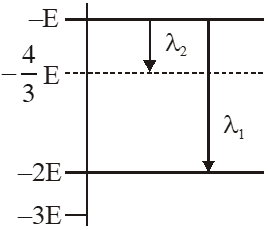(1) $\mathrm{r}=\frac{3}{4}$ (2) $\mathrm{r}=\frac{1}{3}$ (3) $\mathrm{r}=\frac{4}{3}$ (4) $r=\frac{2}{3}$ [JEE Main-2017]
Ans. (2) using $\Delta \mathrm{E}=\frac{\mathrm{hC}}{\lambda}$ for $\lambda_{1} \quad-\mathrm{E}-(-2 \mathrm{E})=\frac{\mathrm{hC}}{\lambda_{1}}$ $\lambda_{1}=\frac{\mathrm{hC}}{\mathrm{E}}$ for $\lambda_{2} \quad-\mathrm{E}-\left(-\frac{4 \mathrm{E}}{3}\right)=\frac{\mathrm{hC}}{\lambda_{2}}$ $\lambda_{2}=\frac{3 \mathrm{h} \mathrm{C}}{\mathrm{E}}$ $\frac{\lambda_{1}}{\lambda_{2}}=\mathrm{r}=\frac{1}{3}$
Q. A particle A of mass m and initial velocity v collides with a particle B of mass which is at rest. The collision is head on, and elastic. The ratio of the de–Broglie wavelengths $\lambda_{\mathrm{A}}$ to $\lambda_{\mathrm{B}}$after the collision is : (1) $\frac{\lambda_{\mathrm{A}}}{\lambda_{\mathrm{B}}}=\frac{2}{3}$ (2) $\frac{\lambda_{\mathrm{A}}}{\lambda_{\mathrm{B}}}=\frac{1}{2}$ (3) $\frac{\lambda_{\mathrm{A}}}{\lambda_{\mathrm{B}}}=\frac{1}{3}$ (4) $\frac{\lambda_{\mathrm{A}}}{\lambda_{\mathrm{B}}}=2$   [JEE Main-2017]
Ans. (4)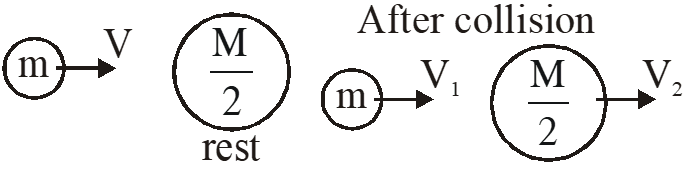By conservation of linear momentum $\mathrm{mv}=\mathrm{mv}_{1}+\frac{\mathrm{m}}{2} \mathrm{v}_{2}$ $2 \mathrm{v}=2 \mathrm{v}_{1}+\mathrm{v}_{2}$ ....(1) by law of collision $\mathrm{e}=\frac{\mathrm{V}_{2}-\mathrm{V}_{1}}{\mathrm{u}_{1}-\mathrm{u}_{2}}$ $\mathrm{u}=\mathrm{v}_{2}-\mathrm{v}_{1}$ ...(2) By equation (1) and (2)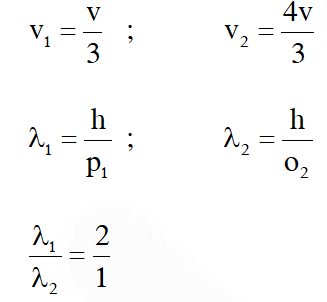Option (4)
Q. An electron from various excited states of hydrogen atom emit radiation to come to the ground state. Let $\lambda_{\mathrm{n}}, \lambda_{\mathrm{g}}$ be the de Broglie wavelength of the electron in the nth state and the ground state respectively. Let be the wavelength of the emitted photon in the transition from the nth state to the ground state. For large n, (A, B are constants) $(1) \Lambda_{\mathrm{n}} \approx \mathrm{A}+\mathrm{B} \lambda_{\mathrm{n}}$ $(2) \Lambda_{\mathrm{n}}^{2} \approx \mathrm{A}+\mathrm{B} \lambda_{\mathrm{n}}^{2}$ (3) $\Lambda_{\mathrm{n}}^{2} \approx \lambda$ (4) $\Lambda_{\mathrm{n}} \approx \mathrm{A}+\frac{\mathrm{B}}{\lambda_{\mathrm{n}}^{2}}$ [JEE Main-2018]
Ans. (4) $\lambda_{\mathrm{n}}=\frac{\mathrm{h}}{\mathrm{mu}}=\frac{\mathrm{h}}{\sqrt{2 \mathrm{mk}_{\mathrm{n}}}}$ $\Rightarrow \mathrm{k}_{\mathrm{n}}=\frac{\mathrm{h}^{2}}{2 \mathrm{m} \lambda_{\mathrm{n}}^{2}} ; \mathrm{k}_{\mathrm{g}}=\frac{\mathrm{h}^{2}}{2 \mathrm{m} \lambda_{\mathrm{g}}^{2}}$ $\Rightarrow \mathrm{k}_{\mathrm{g}}-\mathrm{k}_{\mathrm{n}}=\frac{\mathrm{h}^{2}}{2 \mathrm{m}}\left[\frac{1}{\lambda_{\mathrm{g}}^{2}}-\frac{1}{\lambda_{\mathrm{n}}^{2}}\right]$ $\mathrm{E}_{\mathrm{n}}=-\mathrm{k}_{\mathrm{n}}$ for emitted photon $\frac{\mathrm{hc}}{\Lambda_{\mathrm{n}}}=\mathrm{E}_{\mathrm{n}}-\mathrm{E}_{\mathrm{g}}=\mathrm{K}_{\mathrm{g}}-\mathrm{K}_{\mathrm{n}}$ $\frac{1}{\Lambda_{\mathrm{n}}}=\frac{\mathrm{K}_{\mathrm{g}}-\mathrm{K}_{\mathrm{n}}}{\mathrm{hc}}$ $\Lambda_{\mathrm{n}}=\frac{\mathrm{hc}}{\mathrm{K}_{\mathrm{g}}-\mathrm{K}_{\mathrm{n}}} \Rightarrow \Lambda_{\mathrm{n}}=\frac{\mathrm{hc}}{\frac{\mathrm{h}^{2}}{2 \mathrm{m}}\left[\frac{1}{\lambda_{\mathrm{g}}^{2}}-\frac{1}{\lambda_{\mathrm{n}}^{2}}\right]}$ $\Lambda_{\mathrm{n}}=\frac{2 \mathrm{mc}}{\mathrm{h}\left(\frac{\lambda_{\mathrm{n}}^{2}-\lambda_{\mathrm{g}}^{2}}{\lambda_{\mathrm{g}}^{2} \lambda_{\mathrm{n}}^{2}}\right)}$ $\Lambda_{\mathrm{n}}=\frac{2 \mathrm{mc} \lambda_{\mathrm{g}}^{2} \lambda_{\mathrm{n}}^{2}}{\mathrm{h}\left(\lambda_{\mathrm{n}}^{2}-\lambda_{\mathrm{g}}^{2}\right)}$ as $\lambda_{\mathrm{n}} \propto \mathrm{n}$ $\lambda_{\mathrm{n}}>>\lambda_{\mathrm{g}}$ $\Lambda_{\mathrm{n}}=\frac{2 \mathrm{mc} \lambda_{\mathrm{g}}^{2}}{\mathrm{h}}\left[1-\left(\frac{\lambda_{\mathrm{g}}}{\lambda_{\mathrm{n}}}\right)^{2}\right]^{-1}$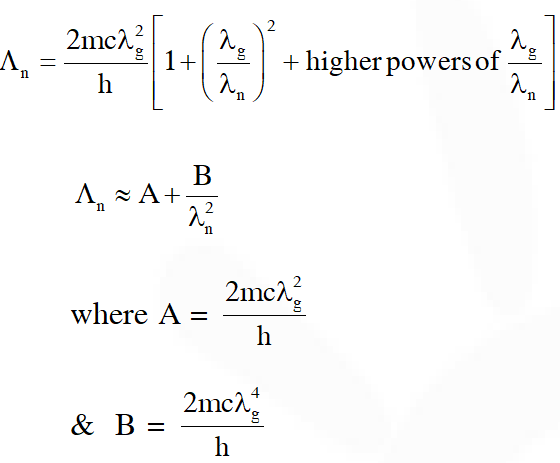Q. If the series limit frequency of the Lyman series is $\mathrm{v}_{\mathrm{L}}$, then the series limit frequency of the Pfund series is : (1) $16 \mathrm{v}_{\mathrm{L}}$ (2) $\mathrm{v}_{\mathrm{L}} / 16$ (3) $\mathrm{v}_{\mathrm{L}} / 25$ (4) $25 \mathrm{v}_{\mathrm{L}}$ [JEE Main-2018]
Ans. (3)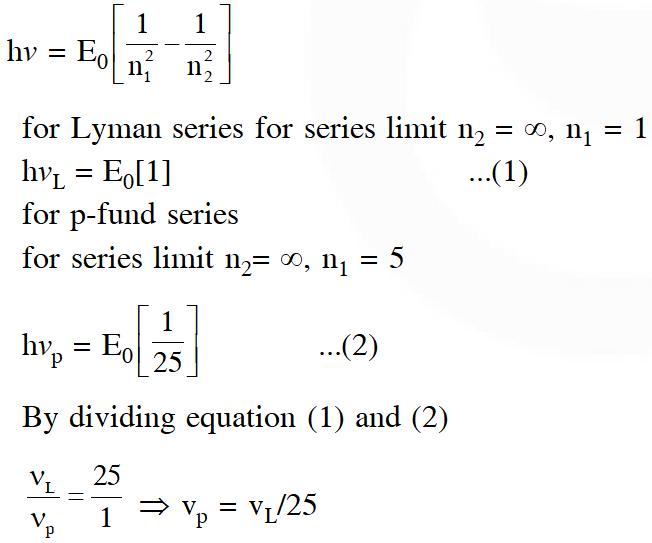VECTOR THARUN
June 17, 2021, 12:16 p.m.
Sir the last question, Answer is Opt D
mansvi
April 21, 2021, 3:18 p.m.
thanks
Sai
Feb. 22, 2021, 11:19 a.m.
They can't make me any small help
Sai
Feb. 22, 2021, 11:19 a.m.
Jan. 17, 2021, 8:08 p.m.
REALLY Helped a lot....thank you &lt;3
Nitheesh
Nov. 11, 2020, 3:42 p.m.
Nihith
Nov. 11, 2020, 3:40 p.m.
Sanjay
Sept. 6, 2020, 9:07 a.m.
Thank u sooooooooo much😍😍😍😍👌🏿👌🏿
Aug. 31, 2020, 12:33 p.m.
Ye sala sarcastically bol raha hai 😠 Dhund ke maaro isko
alok kumar gothwal
Aug. 25, 2020, 1:12 p.m.
very nice
Aman Shetty
Aug. 15, 2020, 10:36 a.m.
Thank you very much esaral
O P Sharma
July 17, 2020, 11:10 p.m.
Very helpful for the aspiring students. Great service to the nation and humanity.
Mayur
July 6, 2020, 11:58 p.m.
Thanks
Jeevan
June 18, 2020, 7:29 a.m.
Guys nice effort but there are some problems yet they are the solution is not clearly understood
Pavan
May 7, 2020, 9:09 p.m.
Thanks